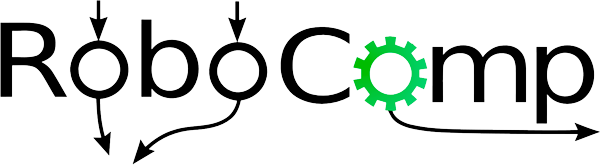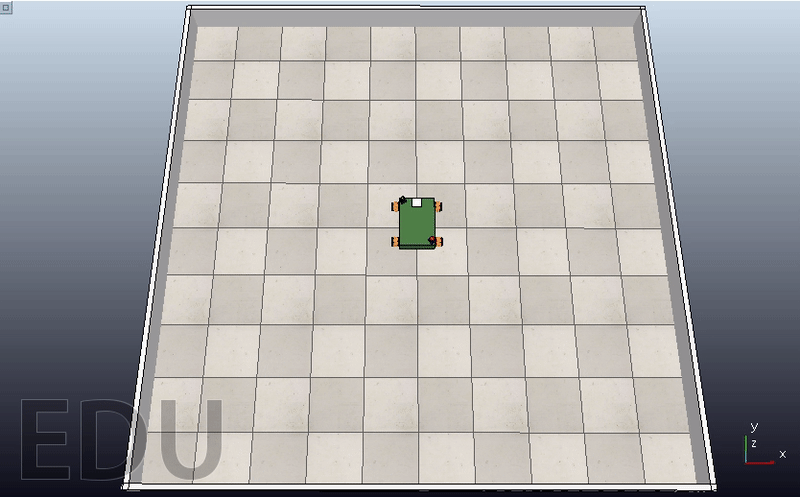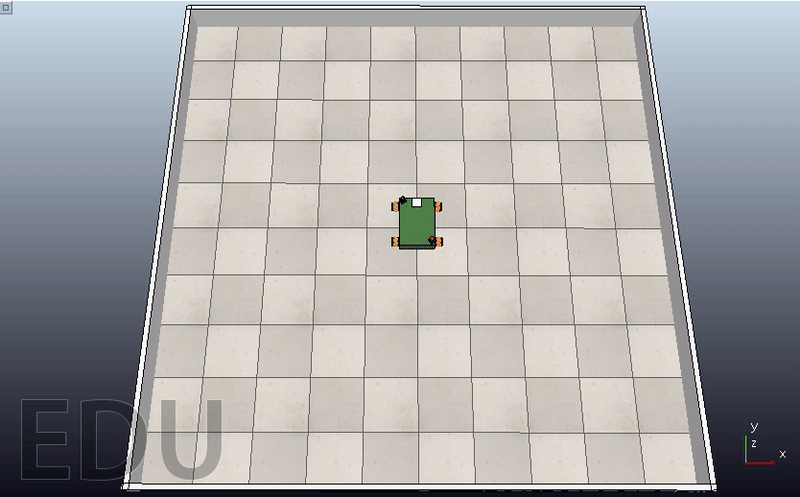# GSoC’21 RoboComp project: Simultaneous path planning and following using Model Predictive Control (SPAF)

19th August, 2021

# Differential Robot

Many mobile robots use differential drive mechanism in their motion. By definition, it’s simplest form consisting of two wheels mounted on a common axis, where each wheel must rotate about a point lying on it know as the Instantaneous Center of Curvature (ICC), and each wheel can be driven independently in a direction. The equations of the velocity for each side (right and left) can be described as follows:

Knowing that “w” is the angular velocity, “R” the signed distance from the ICC to the midpoint between the wheels, and finally “l” is the distance between the centers of the two wheels.

Moreover, by studying the equation with respect to “R” and “w”, we can deduce three senarios:

• When the two velocities are equal in magnitude and direction, the robot will move linearly (R tends to infinity).
• When the two velocities are equal in magnitude but with opposite direction, the robot will rotate in place.
• When only one velocity is equal to zero, the robot will rotate about one side wheel.

To switch between the omni-directional drive mode and the differential drive mode, a flag is raised. Intuitively, it sets the lower and upper bounds of the normal velocity component of the robot (i.e., Vx the velocity in X-direction) by zero.

        if isDifferential:
args['lbx'][h_states: h_states+h_controls: n_controls+1] = 0
args['ubx'][h_states: h_states+h_controls: n_controls+1] = 0


This also can be proved mathematically. In order to satisfy the mecanum wheeled robot’s inverse kinematic model, the trick lies on assuming two wheels instead of four. This will leads us to the same condition for the boundries.

        # calculate mpc in world frame
controlMPC = self.controller.compute(initialState, targetState, controlState, isDifferential=True)


# The Simulation

By giving the robot a point [1200,1800,0.5pi] as a target to compare the motion between differential mode and omni-directional mode.

1. Differential Mode:1. Omni-directional Mode: